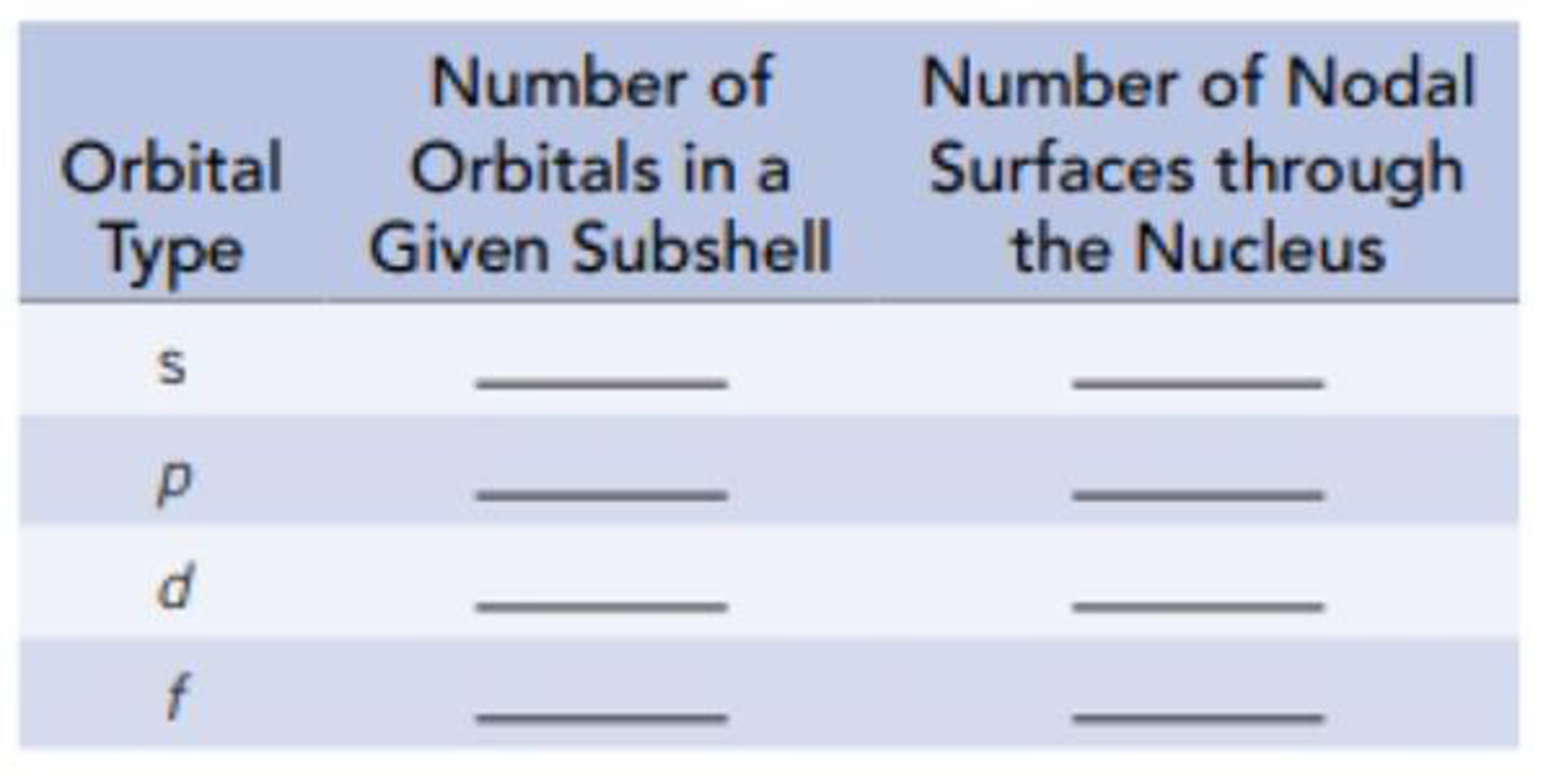Chapter 6, Problem 53GQ

Chapter
Section
Textbook Problem

Complete the following table.Interpretation Introduction

Interpretation: The maximum number of orbitals and number of nodal surfaces through the nucleus of s, p, d and f orbitals should be identified.

Concept introduction:

Nodal surfaces: the surface which passing through nucleus where there is zero chance of finding electrons.

The values of l (azimuthal quantum number) when the principal quantum number is n are from 0 to (n1). Each value of l indicates subshell and for l=0,2 and 3 represents s, d and f subshells.

In a subshell there are (2l+1) number of orbital

Explanation
• Each value of l indicates subshell and for s subshells, l=0. In a subshell there are (2l+1) number of orbital Hence, there is only ((2×0)+1)=1 orbital for s subshells. The s subshell has spherical shape and does not have any sharp boundary where the chance of finding electron is zero. The chance of finding electron is never zero in a s subshell. Therefore, zero nodal surface for s orbital
• Each value of l indicates subshell and for p subshells, l=1. In a subshell there are (2l+1) number of orbital. Hence, there are ((2×1)+1)=3 orbitals for p subshells. For p subshells, l=1 and has dumbbell shape. One nodal surface is passing through the p subshell and thus has two electron density regions. Therefore, 1 nodal surfaces for p orbital.
• Each value of l indicates subshell and for d subshells, l=2. In a subshell there are (2l+1) number of orbital. Hence, there are ((2×2)+1)=5 orbitals for d subshells. For d subshells, l=2

Still sussing out bartleby?

Check out a sample textbook solution.

See a sample solution

The Solution to Your Study Problems

Bartleby provides explanations to thousands of textbook problems written by our experts, many with advanced degrees!

Get Started

Find more solutions based on key concepts